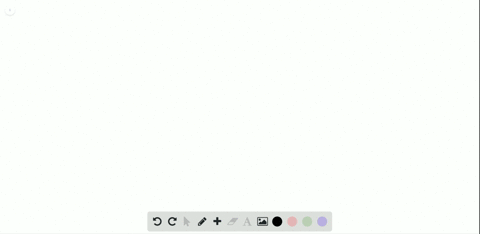Enroll in one of our FREE online STEM bootcamps. Join today and start acing your classes!View Bootcamps### A right circular cone has base of radius 1 and he…

01:51University of Southern California
Problem 85

# Inscribe a rectangle of base $b$ and height $h$ in a circle of radius one, and inscribe an isosceles triangle in a region of the circle cut off by one base of the rectangle (with that side as the base of the triangle). For what value of $h$ do the rectangle and triangle have the same area?

## Discussion

You must be signed in to discuss.

## Video Transcript

So we give it another interesting problem. We're told to inscribe a rectangle of base be based B and high H height age in a circle of radius one in a circle with a radius of one and inscribe. And I saw. So these triangle in a region of the circle cut off by one of the base rectangles by one base of the rectangle and that side eyes to be a basic triangle. For what value of Asia. The rectangle and trying will have the same exact area. Okay, so we know that um the rectangle our will call this. Here we go. The wreck tangle. We could denote by saying s wrecked For the surveyor of the rectangle is equal to base times height, right? And we know that the triangle area Try a gold. Ah, we don't. You know by s Trig is equal to or a stage s try. Let's try. I equal to base times one minus h over too. Over to So the air. The right thing was easy. The area of the triangle is there more tricky. We have the base of the triangle. So if you manage to figure out the height that we can use. The formula of s triangle is equal to base times height over too. So if we draw a line in the center of the rectangle which is also center of the circle to the top of the triangle, the distances are right. This is the radius and ours. You goto what? To get the height of the triangle. We need subtract from the height of the part of the line we drew in the rectangles. That's h over to. Thus, the height of the triangle is one minus h over to which is what I used right here. So we can say at the surface area of the triangle, triangle is able to base times one minus age or two over too. So now that we determine the proper for most of the area, we need to find the value of H for which they're equal. So we're gonna go ahead and solve the obtained equation for age. So we're gonna go ahead and do this on the next page. So we're to say base times height is equal. Do base times one minus h over too. All over too. So we say okay to ages equal do one minus H over too, and to age plus h over to is eagle toe one. Thus, five over to H is equal to one thus ages equal to two over five or 2/5 and that is our answer. H is equal to 2/5.